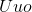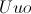# High School Chemistry : Help with Periodic Theories

## Example Questions

### Example Question #1 : Help With Periodic Theories

The periodic table of elements is structured in order of increasing __________.

atomic size

atomic mass

atomic number

electronegativity

ionization energy

atomic number

Explanation:

The periodic table of elements is structured in increasing atomic number. Atomic number of an element refers to the number of protons in an atom of that element. Each element is followed by the next element with one more proton than itself.

Increasing numbers of protons often correspond to atomic mass, but this trend does not hold true in all cases due to variation in isotope variation and neutron addition. For example, the atomic mass of nickel is slightly less than that of cobalt and the atomic mass of hassium is slightly less than that of bohrium.

### Example Question #51 : The Periodic Table

Why does the periodic table of elements end with(ununoctium)?

There are no known cases of atoms which weigh more thanThe periodic table of elements contains hand-picked elements by the Society of Chemistry Scholars (SCS)

None of these answer choices are true

Elements heavier thanare unstable and decay too rapidly to be properly categorizedis the last element that is completely stable

Elements heavier thanare unstable and decay too rapidly to be properly categorized

Explanation:

The periodic table of elements contains the elements that have been deemed as elements by scientists as a collective whole. Although there are atoms with higher masses than, none of them have been stable enough to be considered elements., however, is not completely stable, and complete stability is not a requirement to be considered an element.

### Example Question #53 : The Periodic Table

Why does atomic radius decrease as we move from left to right along a row in the periodic table?

There are more protons in the nucleus to pull in the electrons, reducing the size of the valence shell

The electrons are less stable closer to the nucleus

There are fewer atomic orbitals for the electrons to occupy

There are more neutrons in the nucleus, which makes the protons pull harder on the electrons tor educe the size of the valence shell

There are more atomic orbitals for the electrons to occupy

There are more protons in the nucleus to pull in the electrons, reducing the size of the valence shell

Explanation:

The atomic radius decreases as we move from left to right across a period (row) in the periodic table for a couple reasons. Each time we move from one element to the right, we add a proton and an electron (and a certain number of neutrons depending on the isotope). Because there are no new electron shells present, this extra electron must be added to the same valence shell of this atom. The added positive charge from the proton in the nucleus and the added negative charge from the added electron are opposites, and are thus attracted to each other. The greater the number of each, the greater their pull on one another, and thus the smaller the radius of the atom.

### Example Question #1 : Help With Periodic Theories

The group in the periodic table that is the least chemically reactive is __________.

the transition metals

the halogens

the alkaline earth metals

the noble gases

the alkali metals

the noble gases

Explanation:

The noble gases have a stable octet of electrons in their outer shell and tend to neither lose nor gain electons. They tend to not react with other chemicals.

Alkali metals and halogens are highly reactive; alikali metals need to lose a single electron to reacha a stable octet, while halogens need to gain a single electron. Alkaline earth metals are reactive as well, needing to lose two electrons. Transition metals vary in their valence electron numbers and organizations, but are still considered more reactive than the noble gases.

### Example Question #1 : Help With Periodic Theories

As the atomic number in a period increases, the atomic radii in that period will __________.

increase

The pattern varies with each period

decrease

increase initially, then stay the same

stay the same

decrease

Explanation:

As the atomic number increases in a period, the number of protons increases and creates a stronger electrostatic pull on the electrons. The positive protons in the nucleus attract the negative electrons in the electron cloud. As electrons are added across a pariod, they are added into the same orbital shell (as determined by the principle quantum number of the atom). Since the shell does not change, the radius of the atom does not increase. Rather, the attractive force of the protons acts to decrease the radius of the electron cloud.

### Example Question #56 : The Periodic Table

The use of a periodic table is expected with this question.

In what order would the following elements be if you were to list them from the least amount of protons to the most amount of protons?

Nitrogen, hydrogen, iron, magnesium

Iron, magnesium, nitrogen, hydrogen

Hydrogen, nitrogen, magnesium, iron

Hydrogen, nitrogen, iron, magnesium

Iron, magnesium, hydrogen, nitrogen

Hydrogen, nitrogen, magnesium, iron

Explanation:

The amount of protons in an element is represented by its atomic number. The periodic table is listed in order of atomic number. A smaller atomic number equates to a fewer amount of protons in an element. It is possible to identify the amount of protons for all of the elements listed with the use of a periodic table.

### Example Question #2 : Help With Periodic Theories

Which of the elements listed below has the same number of electrons in the outer shell as calcium?

Barium

Hydrogen

Potassium

Silicon

Neon